# Tetrahedron facts for kids

Kids Encyclopedia Facts

A tetrahedron (triangular pyramid) is a three-dimensional shape. It has four corners. It looks like a pyramid. It has six equally long edges, four corners and four equilateral triangular faces. Every two edges meet on one of those corners forming a sixty-degree angle. All of its sides are triangles.

## Formulas for a regular tetrahedron

A regular tetrahedron is a tetrahedron where all of its edges are the same length. If the length of an edge is a:

 Surface area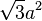${\sqrt{3}}a^2\,$ Face area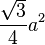$\frac{\sqrt{3}}{4}a^2\,$ Height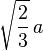$\sqrt{\frac23}\,a\,$ and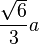$\frac{\sqrt{6}}{3}a$ Volume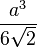$\frac{a^3}{6\sqrt{2}}\,$ and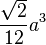$\frac{\sqrt{2}}{12}a^3$

## Images for kidsIn Spanish: Tetraedro para niños